﻿ 多元齐次多项式插值问题研究

# 多元齐次多项式插值问题研究Research on Interpolation of Multivariate Homogeneous Polynomials

Abstract: Based on the basic theory and method of algebraic geometry, this paper studies the problem of multivariate homogeneous polynomial interpolation. In this paper, we obtain the superposition construction method of constructing multivariate homogeneous interpolation polynomial space and interpolation regular node group along multivariate homogeneous algebraic hyper surfaces. The topological structure of the regular node group of multivariate homogeneous interpolation is basically clarified, and an experimental example is given to verify the theoretical method.

1. 引言

2. 基本概念和主要定理

${H}_{n}^{\left(s\right)}=\left\{\underset{{\alpha }_{1}+\cdots +{\alpha }_{s}=n}{\sum }{x}_{1}^{{\alpha }_{1}}{x}_{2}^{{\alpha }_{2}}\cdots {x}_{s}^{{\alpha }_{s}}|{a}_{{\alpha }_{1}{\alpha }_{2}\cdots {\alpha }_{s}}\in R\right\}$

${d}_{n}^{\left(s\right)}=\left(\begin{array}{l}n+s-1\\ \text{}s-1\end{array}\right)$$A={\left\{{Q}_{i}\right\}}_{i=1}^{{d}_{n}^{\left(s\right)}}$${R}^{s}$ 中的 ${d}_{n}^{\left(s\right)}$ 个相异点，任意给定一个实数组 ${\left\{{f}_{i}\right\}}_{i=1}^{{d}_{n}\left(s\right)}$，找到一个多项式 $p\left(x\right)\in {H}_{n}^{\left(s\right)}$ 那么这个多项式需要适合以下的插值条件：

$p\left({Q}_{i}\right)={f}_{i},\text{}i=1,\cdots ,{d}_{n}^{\left(s\right)}$ (1)

${d}_{n}^{\left(s\right)}\left(k\right)=\left(\begin{array}{l}n+s-1\\ \text{}s-1\end{array}\right)-\left(\begin{array}{l}n+s-k-1\\ \text{}s-1\end{array}\right)$ (2)

$h\left({Q}_{i}\right)={f}_{i},\text{}i=1,2,\cdots ,{d}_{n}^{\left(s\right)}\left(k\right)$ (3)

3. 定理的证明

$V\left({I}_{1}\right)\subset V\left({I}_{2}\right)$$I\left(V\left({I}_{1}\right)\right)\supset I\left(V\left({I}_{2}\right)\right)$

$I\left(V\left({I}_{1}\right)\right)=\sqrt{{I}_{1}}={I}_{1}$$I\left(V\left({I}_{2}\right)\right)=\sqrt{{I}_{2}}\supset {I}_{2}$${I}_{1}\supset {I}_{2}$

${h}_{n+k}^{\left(s\right)}=q\left(x\right)r\left(x\right)$ (4)

$A$${H}_{n}^{\left(s\right)}$ 的正则插值结点组， $r\left(x\right)\in {H}_{n}^{\left(s\right)}$ 这显然与 $A$${H}_{n}^{\left(s\right)}$ 的正则插值结点组矛盾。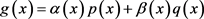(5)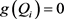,(6)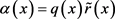(7)

4. 具体构造方法及实验算例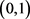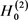的一个齐次正则插值结点组，不经过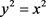上任取两个点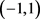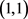，则由定理1知这三点构成的齐次正则插值结点组。取被插值函数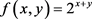，则所确定的唯一一条插值函数，由MATLAB  做出被插值函数与所求二元齐次插值多项式的图像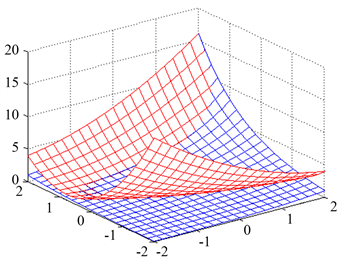Figure 1. Effect diagram of bivariate homogeneous interpolation

 梁学章. 二元插值的适定结点组与迭加插值法[J]. 吉林大学学报(自然科学版), 1979(1): 27-32.

 梁学章, 李强. 多元逼近[M]. 北京: 国防工业出版社, 2005: 22-28.

 崔利宏, 杨一浓, 王晓婉. 平面代数曲线上的二元多项式插值问题研究[J]. 辽宁师范大学学报(自然科学版), 2013(36): 318-321.

 崔利宏, 范晓倩, 刘莹. 多元分次插值适定性问题研究[J]. 辽宁师范大学学报(自然科学版), 2016(12): 433-437.

 崔利宏. 多元Lagrange插值与多元Kergin插值[M]. 大连: 辽宁师范大学出版社, 2018: 36-42.

 周蕴时, 苏志勋, 奚涌江, 等. CAGD中的曲线与曲面[M]. 长春: 吉林大学出版社, 1993.

Top